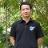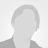# Inter-Domain Path Computation under Domain Uniqueness constraint (IDPCDU) Instances

Published: 18 Jan 2020 | Version 3 | DOI: 10.17632/t726xwcjf9.3
Contributor(s):
• Thanh Pham Dinh,Unspecified
• Thang Ta Bao,Unspecified
Hanoi University of Science & Technology
• Hoang Ngo Viet,Hoang Ngo Viet
Hanoi University of Science & Technology
• Long Nguyen BinhLong Nguyen Binh
Hanoi University of Science & Technology

## Description of this data

• The tested data for Inter-Domain Path Computation under Domain Uniqueness constraint (IDPCDU).

• On account of no instances were available for IDPC-DU, we made up our minds to generate a set for test instances to evaluate the proposed algorithms. To generate an instance, we first passed three parameter: number of nodes, number of domains and number of edges. After that, we created an array of distinct nodes and an array of distinct domains that satisfied the number of nodes is greater than the length of domain array. Source nodes and terminal node are the first and the last nodes of the nodes array, respectively. With the above arrays, we merged them to make a valid solution called P. Each edge of P was set to the weight one, except the out-edge of the source node is set to the weight two. To add noise to the test instance, for every single node in
P, we added some edges to random nodes not in P. Moreover, we created some one-weighed-edges between the the nodes not in P. These traps would make simple greedy algorithms get it harder to find optimal solution. Eventually, we randomly generated edges that have greater values of weight than the value of the length of P. This method guaranteed that P is the optimal solution of the instance. There were two set of instances created, a small set and a large set.

• Filename idpc_<number of nodes>x<number of domain>x<number of edges>.idpc
First line of a file constains two intergers N and D, which are number of nodes and number of domains, respectively.
Second line contains two integers s and t, which are the source node and terminal node.
Every next line contains four integers u, v, w, d, represents an edge (u,v) has weight w and belong to domain d.

• IDPC-DU
Cite

## Steps to reproduce

we made up our minds to generate a set for test instances to evaluate the proposed algorithms. To generate an instance, we first passed three parameter: number of nodes, number of domains and number of edges. After that, we created an array of distinct nodes and an array of distinct domains that satisfied the number of nodes is greater than the length of domain array. Source nodes and terminal node are the first and the last nodes of the nodes array, respectively. With the above arrays, we merged them to make a valid solution called P. Each edge of P was set to the weight one, except the out-edge of the source node is set to the weight two. To add noise to the test instance, for every single node in
P, we added some edges to random nodes not in P. Moreover, we created some one-weighed-edges between the the nodes not in P. These traps would make simple greedy algorithms get it harder to find optimal solution. Eventually, we randomly generated edges that have greater values of weight than the value of the length of P. This method guaranteed that P is the optimal solution of the instance. There were two set of instances created, a small set and a large set.

• ### Version 3

2020-01-18

Published: 2020-01-18

DOI: 10.17632/t726xwcjf9.3

### Cite this dataset

Pham Dinh, Thanh; Ta Bao, Thang; Ngo Viet, Hoang; Nguyen Binh, Long (2020), “Inter-Domain Path Computation under Domain Uniqueness constraint (IDPCDU) Instances”, Mendeley Data, v3 http://dx.doi.org/10.17632/t726xwcjf9.3

Views: 27

## Compare to version

### Institutions

Hanoi University of Science and Technology

### Categories

Genetic Algorithm, Actuator Networks, Evolutionary Algorithm